Courses

# Simple Stresses (Part - 2) Mechanical Engineering Notes | EduRev

## Mechanical Engineering : Simple Stresses (Part - 2) Mechanical Engineering Notes | EduRev

The document Simple Stresses (Part - 2) Mechanical Engineering Notes | EduRev is a part of the Mechanical Engineering Course Machine Design.
All you need of Mechanical Engineering at this link: Mechanical Engineering

Bending of beams

Bending stresses Consider two sections ab and cd in a beam subjected to a pure bending. Due to bending the top layer is under compression and the bottom layer is under tension. This is shown in figure-2.1.3.1.1. This means that in between the two extreme layers there must be a layer which remains un-stretched and this layer is known as neutral layer. Let this be denoted by NN′.Bending of beams 2.1.3.1 Bending stresses Consider two sections ab and cd in a beam subjected to a pure bending. Due to bending the top layer is under compression and the bottom layer is under tension. This is shown in figure-2.1.3.1.1. This means that in between the two extreme layers there must be a layer which remains un-stretched and this layer is known as neutral layer. Let this be denoted by NN′.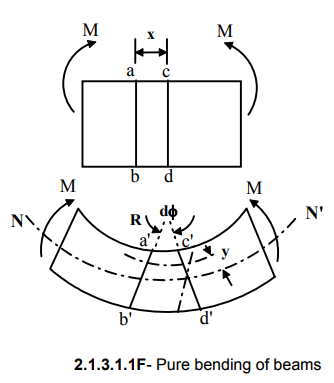We consider that a plane section remains plane after bending- a basic assumption in pure bending theory.
If the rotation of cd with respect to ab is dφ the contraction of a layer y distance away from the neutral axis is given by ds=y dφ and original length of the layer is x=R dφ, R being the radius of curvature of the beam. This gives the strain ε in the layer as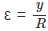We also consider that the material obeys Hooke’s law σ = Eε. This is another basic assumption in pure bending theory and substituting the expression for ε we have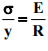Consider now a small element dA y distance away from the neutral axis. This is shown in the figure 2.1.3.1.2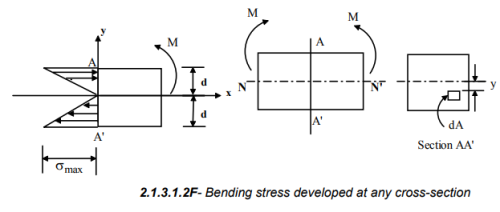Axial force on the element d  dFσx= dA    and considering the linearity in stress variation across the section :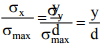we have where σx and σmax are the stresses at distances y and d respectively from the neutral axis. The axial force on the element is thus given by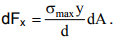This gives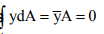and since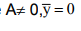This means that the neutral axis passes through the centroid. Again for static equilibrium total moment about NA must the applied moment M. This is given by and this gives   σmax =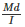For any fibre at a distance of y from the centre line we may therefore write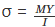We therefore have the general equation for pure bending as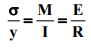Shear stress in bending In an idealized situation of pure bending of beams, no shear stress occurs across the section. However, in most realistic conditions shear stresses do occur in beams under bending. This can be visualized if we consider the arguments depicted in figure-2.1.3.2.1 and 2.1.3.2.2.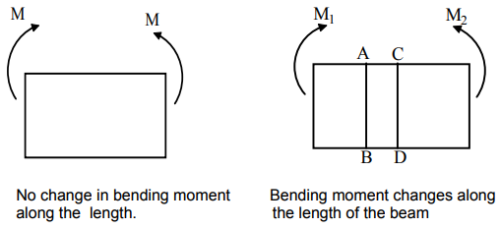2.1.3.2.1F- Bending of beams with a steady and varying moment along its length.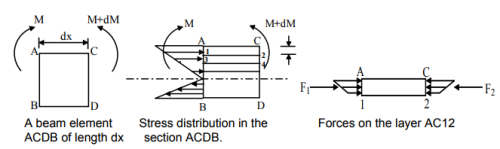2.1.3.2.2F- Shear stress developed in a beam subjected to a moment varying along the length

When bending moment changes along the beam length, layer AC12 for example, would tend to slide against section 1243 and this is repeated in subsequent layers. This would cause interplanar shear forces Fand Fat the faces A1 and C2 and since the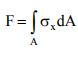force at any cross-section is given by , we may write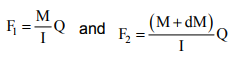Here M and dM are the bending moment and its increment over the length dx and Q is the 1st moment of area about the neutral axis. Since shear stress across the layers can be given by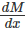and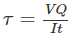shear force is given by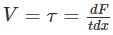we may write

Torsion of circular members

A torque applied to a member causes shear stress. In order to establish a relation between the torque and shear stress developed in a circular member, the following assumptions are needed:

1. Material is homogeneous and isotropic
2. A plane section perpendicular to the axis of the circular member remains plane even after twisting i.e. no warping.
3. Materials obey Hooke’s law.

Consider now a circular member subjected to a torque T as shown in figure 2.1.4.1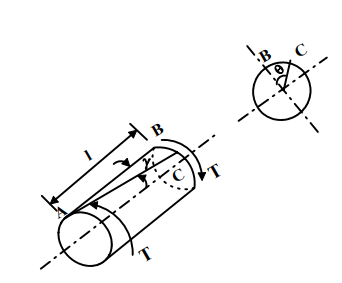2.1.4.1F- A circular member of radius r and length L subjected to torque T.

The assumption of plane section remaining plane assumes no warping in a circular member as shown in figure- 2.1.4.2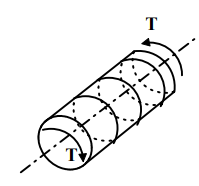2.1.4.2F- Plane section remains plane- No warping.

However, it has been observed experimentally that for non-circular members warping occurs and the assumption of plane sections remaining plane does not apply there. This is shown in figure-2.1.4.3.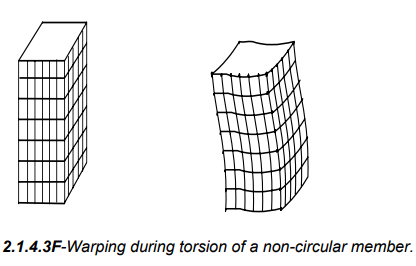Let the point B on the circumference of the member move to point C during twisting and let the angle of twist be θ. We may also assume that strain γ varies linearly from the central axis. This gives

γ = rθ and from Hooke's law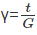where τ is the shear stress developed and G is the modulus of rigidity. This gives

•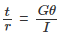Consider now, an element of area dA at a radius r as shown in figure-2.1.4.4. The torque on the element is given by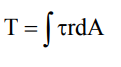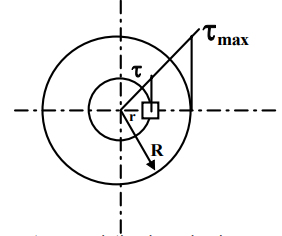2.1.4.4F- Shear stress variation in a circular cross-section during torsion.

For linear variation of shear stress we have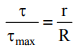Combining this with the torque equation we may write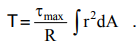Now ∫r2 dA may be identified as the polar moment of inertia J .

And this gives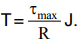Therefore for any radius r we may write in general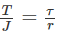We have thus the general torsion equation for circular shafts as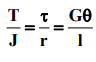Buckling

The compressive stress of P/A is applicable only to short members but for long compression members there may be buckling, which is due to elastic instability. The critical load for buckling of a column with different end fixing conditions is given by Euler’s formula ( figure-2.1.5.1)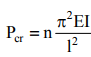where E is the elastic modulus, I the second moment of area, l the column length and n is a constant that depends on the end condition. For columns with both ends hinged n=1, columns with one end free and other end fixed n=0.25, columns with one end fixed and other end hinged n=2, and for columns with both ends fixed n=4.Offer running on EduRev: Apply code STAYHOME200 to get INR 200 off on our premium plan EduRev Infinity!

## Machine Design

57 videos|71 docs|102 tests

,

,

,

,

,

,

,

,

,

,

,

,

,

,

,

,

,

,

,

,

,

;